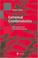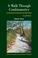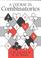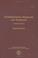### Extremal Combinatorics

#### Course Description

Applications of classical combinatorics for extremal problems in discrete mathematics. Extremal set theory. Fragments of Ramsey's theory. The probabilistic method. The linear algebra method.

#### General Competencies

Fundamental knowledge of classical combinatorics with applications for extremal problems in discrete mathematics. Fragments of extremal set theory, Ramsey theory, as well as two recent methods: the probabilistic method and the linear algebra method. Experience in linking different areas of mathematics (combinatorics, probability and linear algebra) and applying recent mathematical techniques with striking applications in computer science.

#### Learning Outcomes

1. analyze various combinatorial identities.
2. apply the basics of classical combinatorics to solve extremal problems.
3. identify the theory and some applications of extremal set theory.
4. recognize the fundamentals and some applications of Ramsey theory.
5. recognize the fundamentals and some applications of the probabilistic method.
6. recognize the fundamentals and some applications of the linear algebra method.

#### Forms of Teaching

Lectures

Lectures are organized through two cycles. The first cycle consists of 6 weeks of classes and mid-term exam, a second cycle of 7 weeks of classes and final exam. Classes are conducted through a total of 15 weeks with a weekly load of 3 hours.

Exams

Mid-term exam in the 7th week of classes and final exam in the 15th week of classes.

Consultations

Consultations are held one hour weekly according to arrangement with students.

Other Forms of Group and Self Study

Solving homework assignment

Continuous Assessment Exam
Homeworks 0 % 10 % 0 % 10 %
Mid Term Exam: Written 0 % 45 % 0 %
Final Exam: Written 0 % 45 %
Exam: Written 0 % 90 %

#### Week by Week Schedule

1. CLASSICAL COMBINATORICS. Basic concepts of combinatorics. The binomial theorem. Identities involving binomial coefficients. Duble counting. Asimptotic formula for binomial coefficients. Binomial coefficients for real numbers. The multionomial theorem.
2. Distribution of "n" objects in "k" boxes. Weak composition of "n". Composition of "n". Set partitions. Stirling numbers of the second kind. Bell number. Integer partitions.
3. Permutations. Cycles in permutations. Number of n-permutations with a given number of cycles. Stirling numbers of the first kind. Connection between Stirling numbers of the first and Stirling numbers of the second kind. Handshaking lemma. Euler's theorem. Turan's number.
4. The averaging principle. Jensen's inequality and applications for the binary trees and prefix-free codes. Bounds on intersection size. The principle of inclusion and exclusion for sets and for arbitrary set functions. Application for the derangements. Application for the Stirling numbers of the second kind.
5. The pigeonhole principle. Some applications for graphs. Erdos-Szekeres theorem. Mantel's theorem. Turan's theorem. Dirichlet's theorem of rational approximations of irrational numbers. The weight shifting argument.
6. Systems of distinct representatives. Hall's marriage theorem. Application for Latin rectangles. Application for decomposition of doubly stohastic matrices. Min-max theorems.
7. Mid-term exam.
8. EXTREMAL SET THEORY. Sunflower lemma. Modifications: relaxed core, relaxed disjointness. Application for the number of minterms of a Boolean function. Application for small depth formulas.
9. Intersecting families. The Erdos-Ko-Rado theorem. Finite ultrafilters. Maximal intersectiong families. A Helly type result.
10. Posets. Chains and antichains. Decomposition of posets. Symmetric chains in power sets. Application for memory allocation problem. Sperner's theorem for antichains in power sets. LYM inequality. Bollobas's theorem.
11. FRAGMENTS OF RAMSEY THEORY. Colorings and Ramsey numbers. Ramsey's theorems for finite graphs. Generalizations. Ramsey's theorem for sets. Shur's theorem. Geometric applications: Erdos-Szekeres theorem for convex polygons and some applications in combinatorial geometry.
12. FRAGMENTS OF THE PROBABILISTIC METHOD. Applications of counting sieve: for Ramsey numbers, Van der Waerden's theorem, tournaments, 2-edge coloring of bipartite graphs, 2-coloring of hypergraphs, Erdos-Ko-Rado theorem.
13. Applications of linearity of expectation: Hamilton paths in tournaments, graphs splitting, sum-free sets, the independence number of a graph. Probability inclusion–exclusion principle. The second moment method: estimating the middle binomial coefficient. Treshold for cliques. Alterations and applications of Markov's inequality.
14. FRAGMENTS OF THE LINEAR ALGEBRA METHOD. Spaces of incidence vectors. Fisher's inequality. Spaces of polynomials. Two-distance sets. Combinatorics of linear spaces. Orthogonality and rank arguments.
15. Final exam.

#### Study Programmes

Computer Engineering (profile)
Mathematics and Science (1. semester)
Computer Science (profile)
Mathematics and Science (1. semester)
Control Engineering and Automation (profile)
Mathematics and Science (1. semester)
Electrical Engineering Systems and Technologies (profile)
Mathematics and Science (1. semester)
Electrical Power Engineering (profile)
Mathematics and Science (1. semester)
Electronic and Computer Engineering (profile)
Mathematics and Science (1. semester)
Electronics (profile)
Mathematics and Science (1. semester)
Information Processing (profile)
Mathematics and Science (1. semester)
Software Engineering and Information Systems (profile)
Mathematics and Science (1. semester)
Telecommunication and Informatics (profile)
Mathematics and Science (1. semester)
Wireless Technologies (profile)
Mathematics and Science (1. semester)

#### LiteratureStasys Jukna (2001.), Extremal Combinatorics With Applications in Computer Science, SpringerMiklos Bona (2006.), A Walk Through Combinatorics, World scientific
B. Stechin, V. Baranov (1995.), Extremal Combinatorial Problems and Their Applications, Kluwer Academic PublishersJ. H. van Lint, R. M. Wilson (1992.), A course in combinatorics, Cambridge University PressL. Lovász (2007.), Combinatorial Problems and Exercises, AMS Chelsea Publishing

#### General

ID 72538
Winter semester
4 ECTS
L1 English Level
L1 e-Learning
45 Lectures